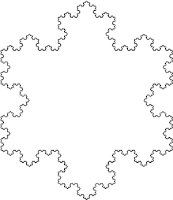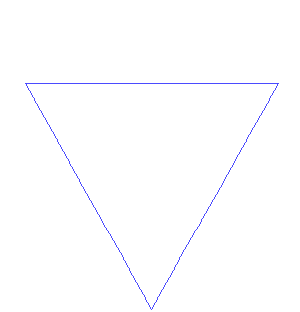## Posts

Showing posts from January, 2012

### Fractal Laws of information

I have published - as a page -  the Laws of Information ; all of which  I have collected, and derived from the fractal. Like a fractal itself, these laws will/should - over time - grow, develop and form shape .

### Fractal SpeedI have since published in 2023:  What is the maximum speed a fractal can be produced?   The fractal is produced at the fractal processing speed (fractal speed), this is the speed at which a  discernible  fractal  shape can be created. This speed also determines the speed of zoom – or magnification into the fractal. It is also the speed of the fractal – wave. Fractal zoom Fractal speed can be demonstrated by drawing the Koch snowflake freehand. This is rather slow and timely ; a much faster method is with modern computers as shown below. The speed is thus limited by the processing power of production. I have published early entries on the production of the fractal. The average modern computer (in 2011) cannot produce many more than 7 iterations  –  in one view or 'fractal paradigm'  – before the computer crashes. To produce more, or see more, we must zoom – forward and into and fractal. The maximum zoom fractal speed must be 'Maxwell’s'  – speed of light

### Fractal UncertaintyI have since published in 2023:  (Fractal)  Quantum Uncertainty Observing a (Koch Snowflake) fractal (Fig. 1 below) in superposition : p osition, scale and direction (of growth) of any one triangle, is only ever known with reference to another reference point – another measurement or observation – and since there is no reference to be found in this state of ceteris paribus or isolation, there is only absolute uncertainly – of the above. In a previous entry – fractal ceteris paribus – I explained this fractal feature – independent of any knowledge of quantum theory. Can the position be determined - in the above fractal animations?

### Fractal: Wave Particle DualityI have since published in 2023:  This entry is one of a set of entries on the fractal and the strange (quantum-like) nature of them. I use the word quantum because there is no other area of knowledge that comes close to explaining or relating to the discoveries I am making with fractal geometry. Blair 11,03,2013 Wave and particle D uality - and the fractal The below entry is a discovery, not an explanation.  I (intend to) write what I see, and what I expect I have found - I do not pretend to fully understand. Just as the atom can ' weirdly' be described as being both a particle and as a ‘smeared out’ wave at the same time, so too, as I shall demonstrate, can the fractal be described in such a way  - only for the fractal it is not so weird. The fractal demonstrating a (discrete) particle: The fractal is defined by  a pattern, object or shape  repeating or iterating. The Koch Snowflake (below) demonstrates this iterating - the triangle represents the particle.

### Fractal EntanglementThis entry I hope adds to the discussion on quantum entanglement. Fractal Entanglement The fractal at a state of fractal superposition, and in perfect isolation – with no interference from other fractals – may demonstrate the principle of (quantum) entanglement.   The 'general' fractal that I have been using to describe our reality in this blog  is defined as a pattern the same, but different, at all scales' and best demonstrated by the Koch Snowflake (below). The Koch Snowflake fractal differs from reality in that it is not 'same but different' at all scales, but is rather an infinity of 'the same but  same , at all scales'. 'Same same' as there is no interference from other fractals to change the shape of any of the triangles. As the triangles are – in principle – the same; they are – in principle –  'entangled', or coupled, or connected – at all (time*) scales; linked or 'parented' by the original (iteration

### Fractal SuperpositionI have since published in 2023:  The (Koch snowflake) fractal demonstrates superposition: The (Koch Snowflake) fractal at shape equilibrium - assuming no interference from others and in perfect isolation from other fractals - demonstrates and is in a state of superposition. It shows the infinite positions, the 'same' triangles (or particles, information, or the rules) can be - in a cross-section view. Koch Snowflake in Superposition ﻿ ﻿ Fractal zoom: infinity

### Fractal Quantum: Intro

I have since published in 2023:  Update: After some 8 years I have finally completed writing up my fractal discoveries.  https://www.researchgate.net/publication/343999170_Making_Sense_of_Light_and_the_Quantum_by_an_Experiment_on_an_Isolated_Emergent_Fractal https://www.academia.edu/43989373/Making_Sense_of_Light_and_the_Quantum_by_an_Experiment_on_an_Isolated_Emergent_Fractal https://vixra.org/abs/2008.0228 This entry introduces a series of entries on the fractal – and ‘quantum’. fractal-superposition fractal-entanglement fractal-wave-particle-duality fractal-uncertainty Late last year I – briefly – published an entry on the subject of ceteris paribus and the fractal . As an entry it completed a set of (fractal) explanations for – what I think to be – the three main assumptions of economics: rationality, and perfect information the others – it was an entry I had been developing since the beginning of this blog and I always knew it was a deep and special one. For reason